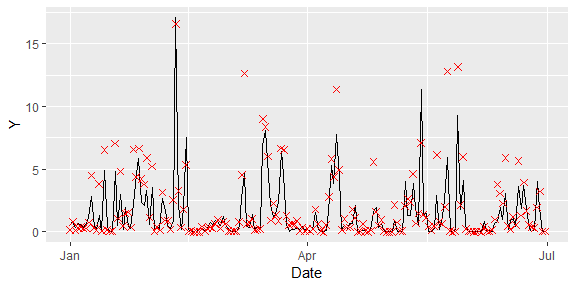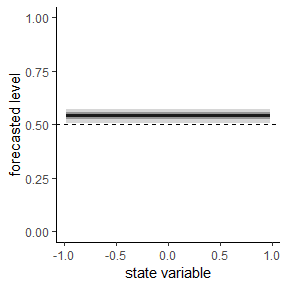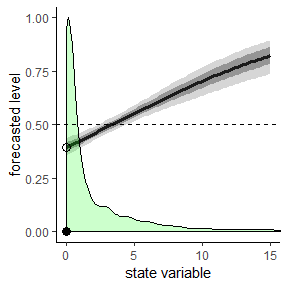# Data


library(PointFore)
library(ggplot2)
library(lubridate)
#>
#> Attaching package: 'lubridate'
#> The following object is masked from 'package:base':
#>
#>     date

precipitation$Date <- as.Date(row.names(precipitation),format = "%d-%m-%Y") ggplot(subset(precipitation, month(Date)< 7 & year(Date)==2013))+ geom_line(aes(x=Date,y=Y))+ geom_point(aes(x=Date,y=X), color = 'red', size = 2, shape=4)Time series of one-day ahead HRES Precipitation forecasts (crosses) and respective observations (solid line) over London. For more information on the data see ?precipitation. # Analysis Now, let us analyse the forecasts. We begin with the constant expectile model.  instruments <- c("lag(lag(Y))","X") res <- estimate.functional(iden.fct = expectiles, model = constant, instruments = instruments, Y = precipitation$Y, X=precipitation$X) #> Drop 2 case(s) because of chosen instruments #> Choose parameter theta0 automatically. summary(res) #>$call
#> estimate.functional(iden.fct = expectiles, model = constant,
#>     Y = precipitation$Y, X = precipitation$X, instruments = instruments)
#>
#> $coefficients #> Estimate Std. Error t value Pr(>|t|) #> Theta 0.5419114 0.020512 26.41924 8.237429e-154 #> #>$Jtest
#>
#>  ##  J-Test: degrees of freedom is 2  ##
#>
#>                 J-test      P-value
#> Test E(g)=0:    3.9107e+01  3.2220e-09
plot(res,hline = TRUE)Constant expectile analysis.

Optimality is rejected with a p-value of 0. On average the forecast tends to overestimation compared to an optimal mean forecast with an expectile level of 0.54.

Next, we consider state-dependent forecasting behavior. Instead of using the conventional state-dependence models we rely on the linear probit specification model but enforce an expectile level of $$0$$ for the forecast $$0$$. This is a logical consequence of precipitation being a positive random variable.

probit0 <- function(stateVariable,theta) probit_linear(stateVariable, theta)*(stateVariable>0)

res <- estimate.functional(iden.fct =   expectiles ,
model = probit0,
theta0 = c(0,0),
instruments = instruments,
state = precipitation$X, Y = precipitation$Y, X=precipitation$X) #> Drop 2 case(s) because of chosen instruments summary(res) #>$call
#> estimate.functional(iden.fct = expectiles, model = probit0, theta0 = c(0,
#>     0), Y = precipitation$Y, X = precipitation$X, stateVariable = precipitation$X, #> instruments = instruments) #> #>$coefficients
#>             Estimate Std. Error   t value     Pr(>|t|)
#> Theta -0.26720297 0.06458326 -4.137341 3.513543e-05
#> Theta  0.08056457 0.01530689  5.263287 1.415021e-07
#>
#> \$Jtest
#>
#>  ##  J-Test: degrees of freedom is 1  ##
#>
#>                 J-test   P-value
#> Test E(g)=0:    0.11280  0.73698

To replicate the result plot in the paper , we need to adjust the standard plot function of the PointFore package to the probit0 specification model.

plot(res,limits = c(0.001,15),hline = TRUE)+
geom_point(data=data.frame(x=c(0,0),y=c(0,.395),shape=c(1,2)),
aes(x=x,y=y,shape=as.factor(shape)),
,size=3,show.legend = FALSE)+
scale_shape_manual(values=c(16,1))Plot linear probit model.